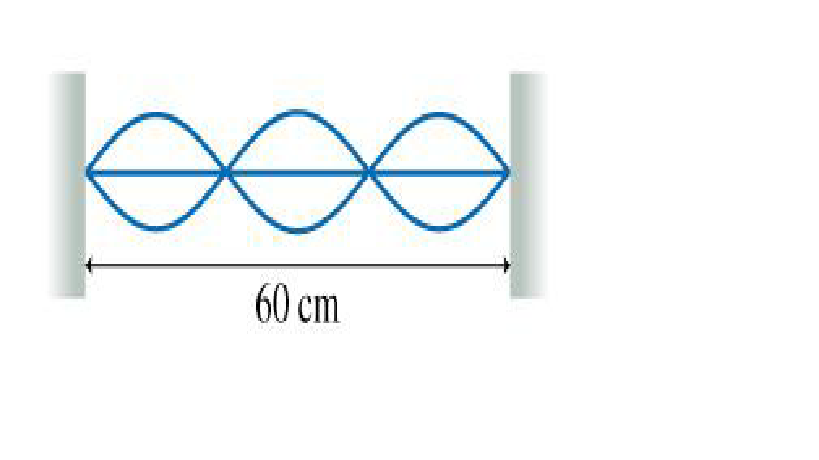# Problem: The figure shows a standing wave oscillating at 100 Hz on a string. What is the wave speed?

###### FREE Expert Solution

Wave speed:

$\overline{){\mathbf{v}}{\mathbf{=}}{\mathbf{f}}{\mathbf{\lambda }}}$

The wavelength and the length of a string are related by the equation:

$\overline{){\mathbf{L}}{\mathbf{=}}\frac{\mathbf{n}\mathbf{\lambda }}{\mathbf{2}}}$, where n is the number of loops.

86% (113 ratings)###### Problem Details

The figure shows a standing wave oscillating at 100 Hz on a string. What is the wave speed?